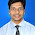Proof :

Suppose f is Riemann integrable on [a, b].
Let $\epsilon>0$ be arbitrary.
$\int\limits_\underline{a}^bf(x)dx=\int\limits_a^\underline{b}f(x)dx$     ..... (1)
By definition of lower integral,
$\int\limits_\underline{a}^bf(x)dx=sup\{L(P, f)$, P is partition of $[a, b]\}$
By definition of supremum,
$\exists$ a partition $P_1$ of [a, b] such that,
$\int\limits_\underline{a}^bfdx-\frac{\epsilon}{2}<L(P_1, f)\leq\int\limits_\underline{a}^bfdx$ ..... (2)
By definition of upper integral,
$\int\limits_a^\underline{b}f(x)dx=inf\{U(P, f)$, P is a partition of $[a, b]\}$
By definition of infimum,
$\exists$ some partition $P_2$ of [a, b] such that,
$\int\limits_a^\underline{b}fdx\leq U(P_2, f)<\int\limits_a^\underline{b}fdx+\frac{\epsilon}{2}$ ..... (3)
Let $P_\epsilon=P_1\cup P_2$ be the refinement of $P_1$ and $P_2$.
$U(P_\epsilon, f)\leq U(P_2, f)$
$L(P_1, f)\leq L(P_\epsilon, f)$
Consider,
$U(P_\epsilon, f)-L(P_\epsilon, f)$
$\leq\int\limits_a^\underline{b}f(x)dx+\frac{\epsilon}{2}-\int\limits_\underline{a}^bf(x)dx+\frac{\epsilon}{2}$ .... from (1), (2) & (3)
$=\epsilon$
Hence, we have partition $P_\epsilon$ such that,
$U(P_\epsilon, f)-L(P_\epsilon, f)<\epsilon$
Conversely,
Let $\epsilon>0$ be arbitrary and for this $\epsilon$,
$\exists$ a partition $P_\epsilon$ such that, $U(P_\epsilon, f)-L(P_\epsilon, f)<\epsilon$.
Now, $U(P_\epsilon, f)\geq L(P_\epsilon, f)$
$\implies 0\leq U(P_\epsilon, f)-L(P_\epsilon, f)<\epsilon$
Since, this inequality true for every $\epsilon>0$,
$\therefore\int\limits_\underline{a}^{b}fdx=\int\limits_a^\underline{b}fdx$
Hence, f is Riemann integrable.

1.1.2.1.